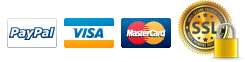# Atlas Basic Construction Math Review, 3rd Edition

\$30.95
##### Vendor: brown-wmarketing

Available

TitleThe Basic Construction Math Review is completely updated and reorganized for current examinations!

Product Details

Construction competency tests are usually book tests. An understanding of formulas, equations, areas and volumes and other math fundamentals is essential if many of the problems on these tests are to be solved correctly.

The Atlas Basic Construction Math Review, 3rd Edition was written to help you learn or re-learn these arithmetic, algebra and geometry fundamentals. Some mathematicians might say that all of the “usual” basic math topics are not covered in the following pages – and they’re right. Only those math basics that will help you pass that competency test are discussed in this manual.

Self-study with any book requires much discipline and diligence. Some time-proven tips have been listed to help you know how to study and gain the most benefit for your time invested. 104pp.

With this manual, you will soon have that good understanding of basic math!

Chapter 1: Fractions

• Reduction of Fractions
• Changing Improper Fractions and Mixed Numbers
• Addition of Fractions and Mixed Numbers
• Subtraction of Fractions and Mixed Numbers
• Multiplication of Fractions and Mixed Numbers
• Cancellation
• Division of Fractions and Mixed Numbers

Chapter 2: Decimals

• Decimal and Fraction Conversion
• Addition and Subtraction of Decimals
• Multiplication of Decimals
• Division of Decimals
• Decimal Multiplication and Division with Tens
• Round Off Decimals

Chapter 3:Inches-Feet Conversions

• Inches To Feet
• Feet To Inches
• Addition and Subtraction of Inches and Feet
• Multiplication and Division of Inches and Feet

Chapter 4: Percentage

• Changing Percent to Decimals
• Changing Percent to Common Fractions
• Changing a Decimal t a Percent
• Changing Common Fractions to Percent
• The Three Types of Percentage Problems

Chapter 5: Ratio

• Roof Pitch

Chapter 6: Proportion

Chapter 7: Algebra Fundamentals

• Subtraction of Signed Numbers
• Multiplication of Signed Numbers
• Division of Signed Numbers
• Parentheses
• Algebraic Expressions

Chapter 8: Equation Solving

• Subtraction of Quantities
• Multiplication of Quantities
• Division of Quantities
• Transposition

Chapter 9: Powers and Roots

Chapter 10: Board Feet

Chapter 11: Areas and Volumes

• Circle
• Triangle
• Right Triangle
• Square
• Rectangle
• Parallelogram
• Trapezoid
• Hexagon
• Octagon
• Cube
• Rectangular Solid
• Cylinder